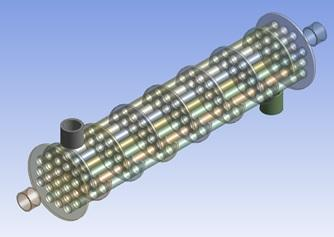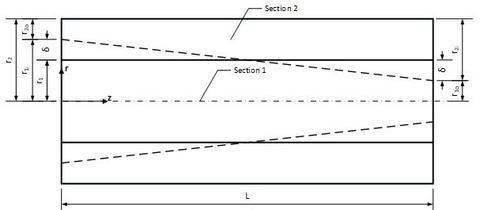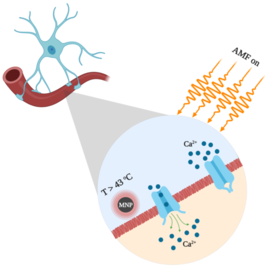# Heat and Mass Transfer

## Current Projects

`Analysis of Nanofluid Flow in Heat Exchangers`A numerical investigation of developing forced convective heat transfer and pressure drop of nanofluid inside tube subjected to constant heat flux boundary condition is presented. The single-phase homogenous and two different two-phase models: Lagrangian-Eulerian model or (discrete phase model) and mixture model are utilized with both constant and temperature-dependent properties to further investigate and clarify the differences and evaluate the assumption of the single-phase model.

`Improvements in Pipe Flow`In this work, we have introduced a new technique to enhance heat transfer utilizing novel configuration improvements. This technique is quite attractive for thermal management of pipe flow especially in applications with limited space. Particularly, we sought to utilize the convergence concept and the wall profile manipulation to analyze the flow and heat transfer improvement, employing conventional and nano-fluids, in innovative convergent pipes and double pipe heat exchangers. We examined the effects of convergence angle, pipe wall profile, the volume fraction of nanoparticles, contraction ratio, as well as Reynolds and Prandtl numbers on the thermal and hydraulic performance of these configurations. Moreover, we computed the performance factor of the enhanced geometry to take into account the heat transfer and pressure drop increments, simultaneously.

`Magnetothermal Stimulation`The study of temperature profiles within the Central Nervous System (CNS) when exposed to an alternative magnetic field as a plausible therapy for neuropsychiatric disorders is crucial. This new procedure can be a better alternative for conventional permanent implanted electrodes treatment for CNS diseases such as Parkinson’s disease. Hyperthermic treatments are highly dependent on biomaterial thermophysical properties, magnetic nanoparticle solution concentration, and magnetic field characteristics. Employing a finite element method to obtain the temperature distribution and ultimately, evaluate the lesion size that the exposed tissue endures is very crucial. The main challenge is to maintain local temperature within the safe domain in which the minimum temperature required for stimulation is 43°C and the upper bound is 50°C to avoid cytotoxicity.

## Previous Projects

• Numerical and Experimental Flow and Heat Transfer in Brake Housing of Aircrafts (Numerical and Experimental)

• Modeling Arterial Transport (Analytical and Numerical)

• Numerical Flow and Heat Transfer in Manufacturing and Processing of Power Electronic Components (Numerical)

• Natural Convection in Open-Ended Configurations (Numerical, Experimental, and Analytical)

• Heat Pipe Analysis and Modeling (Analytical, Numerical and Experimental)

• Cooling of Electronic Components (Numerical, Experimental)

• Jet Impingement and Microchannel Cooling (Analytical and Numerical)

• Fundamental Aspects of Transport through Porous Media (Analytical, Numerical and Experimental)

• Condensation and Phase Change (Numerical and Analytical)

• Multiphase Transport through Porous Media (Analytical and Numerical)

• Heat Transfer and Phase Change Effects on Insulation and Partial Insulations (Numerical and Experimental)

• Comparative Analysis between Various Schemes in Finite Difference and Finite Element (Analytical, Numerical, and Experimental)

• Free Surface Analysis (Analytical, Numerical, and Experimental)

• Buoyancy-Driven Convection (Numerical, Analytical, and Experimental)

• Analysis of High Energy Release/Storage Systems (Numerical and Analytical)

• Melting and Solidification (Numerical)

• Filtration (Numerical)

• Target Design and Modeling for Medical Applications (Analytical, Numerical and Experimental)

• Heat Transfer Regulation and Augmentation (Analytical and Numerical)

• Low-Pressure Turbines

• Flow, heat and mass transfer inside thin films (Analytical and Numerical)

• Control of flow and heat using motivated passive thermal systems (Analytical and Numerical)

• Analysis, control, and enhancements of Bio-detection systems (Analytical and Numerical)

• Land mine detection utilizing thermal analysis methods (Numerical)

• Modeling Bio-medical systems (Analytical and Numerical)

• Dehumidification and regeneration utilizing liquid desiccants (Numerical)

• Modeling and Analysis of Bioheat Transport (Analytical and Numerical)

• Modeling and Analysis of Biofilms (Numerical)

• Modeling and Analysis of PCR Systems (Numerical)

• Modeling and Analysis of Biosensors (Numerical and Analytical)